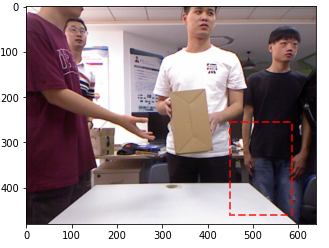Bounding box does not fit the object

I’m doing EDA on the MJU-Waste dataset, and I have found a problem in the visualization of the bounding box for each image. The bounding boxes are in COCO format.

I have tried reading the EXIF orientation tag and rotating the image but it didn’t work. The following image is an example:What else can I try?

For a reason I don’t quite understand and lazily trying a dozen examples or so, it appears as if you would have to multiply the bounding boxes by a factor 0.7 to get the right thing.
Or you could take the polygon annotations and use those.

Note that you should probably link images to annotations via the annotation->image_id and image->id, not go by the indices.

Here is my silly snippet of code:

import json
%matplotlib inline
from matplotlib import pyplot
import PIL.Image
import numpy

ann = {x['image_id']:x for x in d["annotations"]}

idx = 300

im = numpy.array(PIL.Image.open(d["images"][idx]['file_name']))
seg = numpy.array(ann[d["images"][idx]["id"]]['segmentation']).reshape(-1, 2)
bbox = numpy.array(ann[d["images"][idx]["id"]]['bbox'])

x, y, w, h = (numpy.array(bbox) * 0.7).astype(int)
im[y, x:x+w, 0] = 255
im[y+h, x:x+w, 0] = 255
im[y:y+h, x, 0] = 255
im[y:y+h, x+w, 0] = 255

pyplot.figure(figsize=(15, 10))
pyplot.imshow(im)
pyplot.scatter(seg[:, 0], seg[:, 1])

Best regards

Thomas

Thank you Thomas, multiplying the bounding boxes by a factor of 0.7 works really well. Although I think I will compute the bounding boxes from the polygons as you suggested, It seems to be a better solution in the long term.

Thanks!